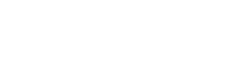Problem: Based on the electronegativities of C, H, and Cl, would you expect the   Cl3CCCH molecule to be  polar or  nonpolar? A molecule is polar if the vector sum of its bond dipole moments is not equal to zero. A molecule is nonpolar if the vector sum of its bond dipole moments is equal to zero. Pauling electronegativity values are listed in the periodic table on the last page of this handout. A. Polar molecule B. Nonpolar molecule

FREE Expert Solution
92% (370 ratings)
Problem Details

Based on the electronegativities of C, H, and Cl, would you expect the   Cl3CCCH molecule to be  polar or  nonpolar? A molecule is polar if the vector sum of its bond dipole moments is not equal to zero. A molecule is nonpolar if the vector sum of its bond dipole moments is equal to zero. Pauling electronegativity values are listed in the periodic table on the last page of this handout.

A. Polar molecule

B. Nonpolar molecule